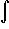#Interactive Real Analysis

Next | Previous | Glossary | Map

## 7.4. Lebesgue Integral

### Example 7.4.4(d): Lebesgue Integral for Simple Functions

Define two simple functions
s1(x) = 2 X[0, 2](x) + 4 X[1, 3](x)
s2(x) = 2 X[0, 1)(x) + 6 X[1, 2](x) + 4 X(2, 3](x)
Show that s1(x) = s2(x) ands1(x) dx =s2(x) dx.
To show that s1(x) = s2(x) is easy:
• Take x[0, 1): Then s1(x) = 2 and s2(x) = 2.
• Take x[1, 2]: Then s1(x) = 2 + 4 = 6 and s2(x) = 6.
• Take x(2, 3]: Then s1(x) = 4 and s2(x) = 4.
Thus the two functions agree.

By definition we have:s1(x) dx = 2 m([0, 2]) + 4 m([1, 3]) = 4 + 8 = 12
ands2(x) dx = 2 m([0, 1)) + 6 m([1, 2]) + 4 m((2, 3]) = 2 + 6 + 4 = 12
so that the value of the integrals agree as well.

In other words, the value of the integral is independent of the representation of the simple functions in this example.

Next | Previous | Glossary | Map